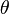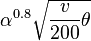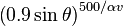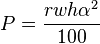# Shield

Shields provide protection against projectiles (Advanced Cannon shells, CRAM Cannon shells, simple weapon shells or fragments) or laser fire. They do not stop missiles, but if the missile gets destroyed before reaching the shield, they will, if the missile has fragmentation warheads, stop the fragments.

## Components

Shields also require power, typically provided by engines.

## Mechanics

### States

This article may need updating to the game's current version. Use information provided here with care.
Reason: "Disrupt and Deflect shields were changed "to be more forgiving of angle of incidence and velocity""

Let$\theta$ be the angle of incidence (i.e. 0 is heading directly into the shield) and the effect strength be$\alpha$.

Shields have four states:

• Off: The shield is inactive, and does not draw any power.
• Disrupt: All projectiles passing through the shield will be deflected by a random angle in each axis up to$\alpha^{0.8} \sqrt{\frac{v}{200} \theta}$

where$\theta$ is the incident angle in degrees.

• Reflect: Projectiles that hit the shield have a chance to be reflected across the shield normal:$\left( 0.9 \sin \theta \right)^{500 / \alpha v}$
• Laser absorb: Absorbs lasers at the cost of 1 energy per damage (in addition to the upkeep cost). The proportion absorbed for an effect strength$\alpha$ is$\frac{100 - 1.66^{10 - \alpha}}{100}$

### Size and position

The projected shield has a rectangular shape. The centre of the shield is always directly in front of the projector. Shields are one-sided, only affecting projectiles coming from the side opposite the generator.

The following settings may be changed:

• Range (1 to 25 m): How far the centre the shield is from the projector.
• Width (1 to 25 m): The width of the shield.
• Height (1 to 25 m): The height of the shield.

### Orientation

• Elevation angle (-45 to 45 degrees): Tilts the shield up and down.
• Azimuth angle (-45 to 45 degrees): Rotates the shield along the axis of projection.

### Effectiveness

The probability of a shield affecting a projectile depends on its effect strength and the incident angle of the projectile.

The effect strength can be set from 1 to 10. Higher values make the shield more likely to affect projectiles.

Projectiles are more likely to be affected if they strike at a glancing angle.

### Power

Shields draw power equal to$P = \frac{r w h \alpha^2}{100}$

where the variables are the range, width, height, and effect strength respectively. The minimum power draw is thus 0.01 and the maximum 15,625.

## Strategy

• For laser absorbing shields, absorption efficiency is maximised at 1.8 effect strength with each such shield absorbing 36.2% of incoming damage. Efficiency is 60.2 dB m3/MW, about three times as efficient as a strength 10 shield. However, it may be worth using a higher strength in order to reduce the number of shield modules.
• It is no longer possible to project shields through other shields, unless placing the shields on different sub-objects